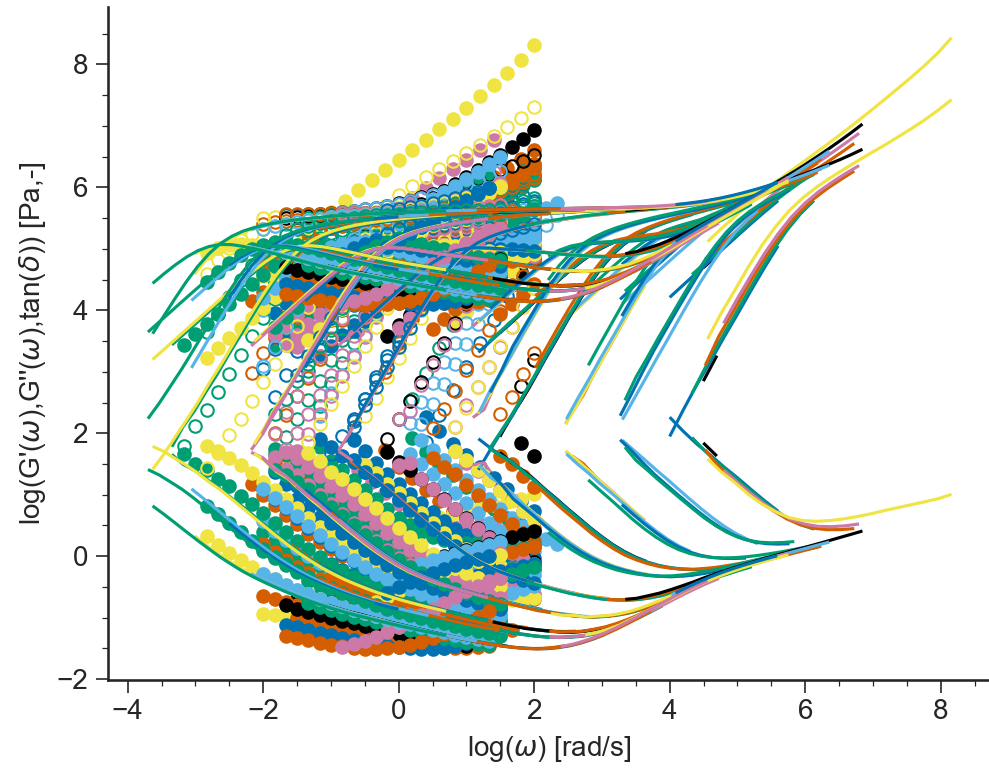# TTS: General description¶

## Purpose¶

Application to Analyze Linear Viscoelastic Data and perform Time-Temperature Superposition

## Data Files¶

• The first line of the file should contain the sample parameters separated by semi-colons (;). It may contain any number of parameters which will be read and saved as file-parameter in RepTate.

• Then the data columns should appear, separated by spaces or tabs.

### .osc extension¶

Text files with .osc extension should be organised as follows:

• .osc files should contaion at least the parameter values for the:

1. sample molar mass Mw,

2. temperature T.

• 3 columns separated by spaces or tabs containing respectively:

1. frequency, $$\omega$$,

2. elastic modulus, $$G'$$,

3. loss modulus $$G''$$.

Other columns will be ingnored. A correct .osc file looks like:

T=0;Mw=94.9;chem=PI;origin=LeedsDA;label=PI88k_09_PP-10;PDI=1.03;
Freq      G'          G"              Temp        Strain
100       3.4801E5    70871       -0.0079     0.96734
68.129    3.328E5     70723       -0.0088     0.96362
46.416    3.1675E5    71696       -0.0101     0.96238
...       ...         ...         ...         ...


## Views¶

### log(G’,G”(w))¶

BaseApplicationTTS.viewLogG1G2()[source]

Logarithm of the storage modulus $$\log(G'(\omega))$$ and loss modulus $$\log(G''(\omega))$$ vs $$\log(\omega)$$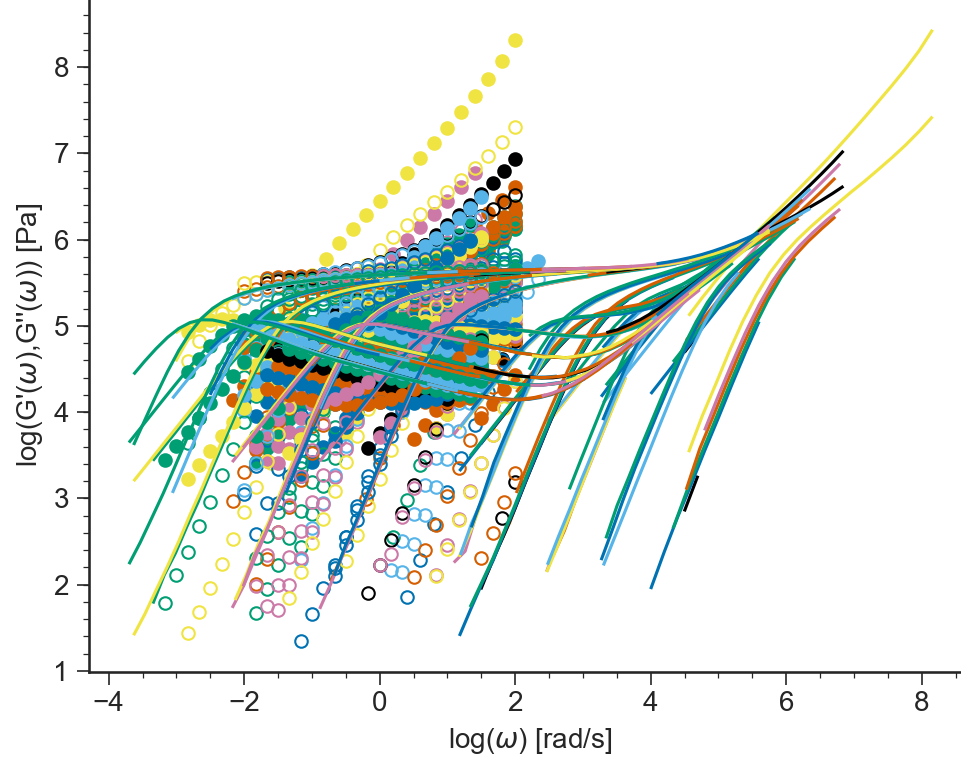### G’,G”(w)¶

BaseApplicationTTS.viewG1G2()[source]

Storage modulus $$G'(\omega)$$ and loss modulus $$G''(\omega)$$ (in logarithmic scale) vs $$\omega$$ (in logarithmic scale)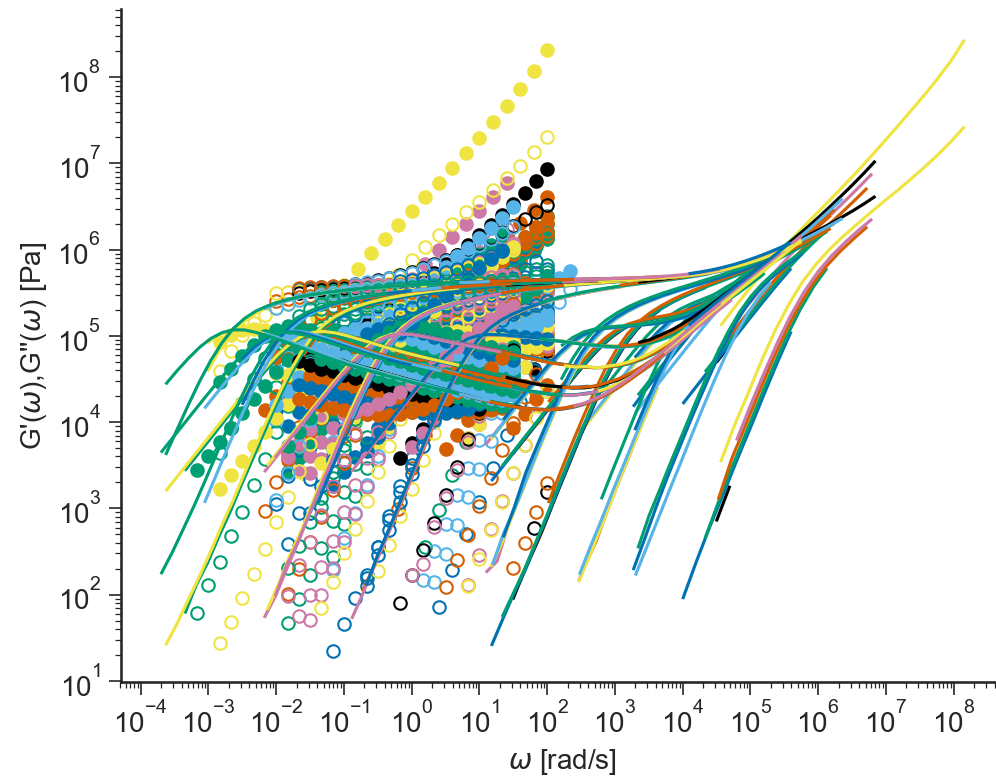### etastar¶

BaseApplicationTTS.viewEtaStar()[source]

Complex viscosity $$\eta^*(\omega) = \sqrt{G'^2 + G''^2}/\omega$$ (in logarithmic scale) vs $$\omega$$ (in logarithmic scale)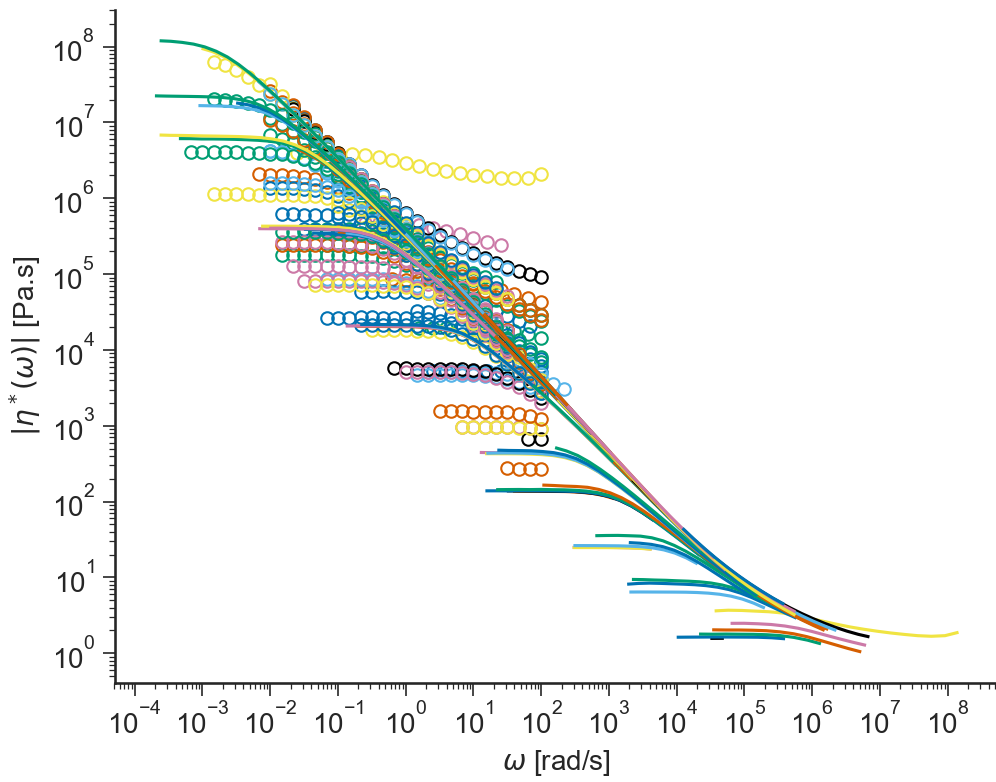### logetastar¶

BaseApplicationTTS.viewLogEtaStar()[source]

Logarithm of the complex viscosity $$\eta^*(\omega) = \sqrt{G'^2 + G''^2}/\omega$$ vs $$\log(\omega)$$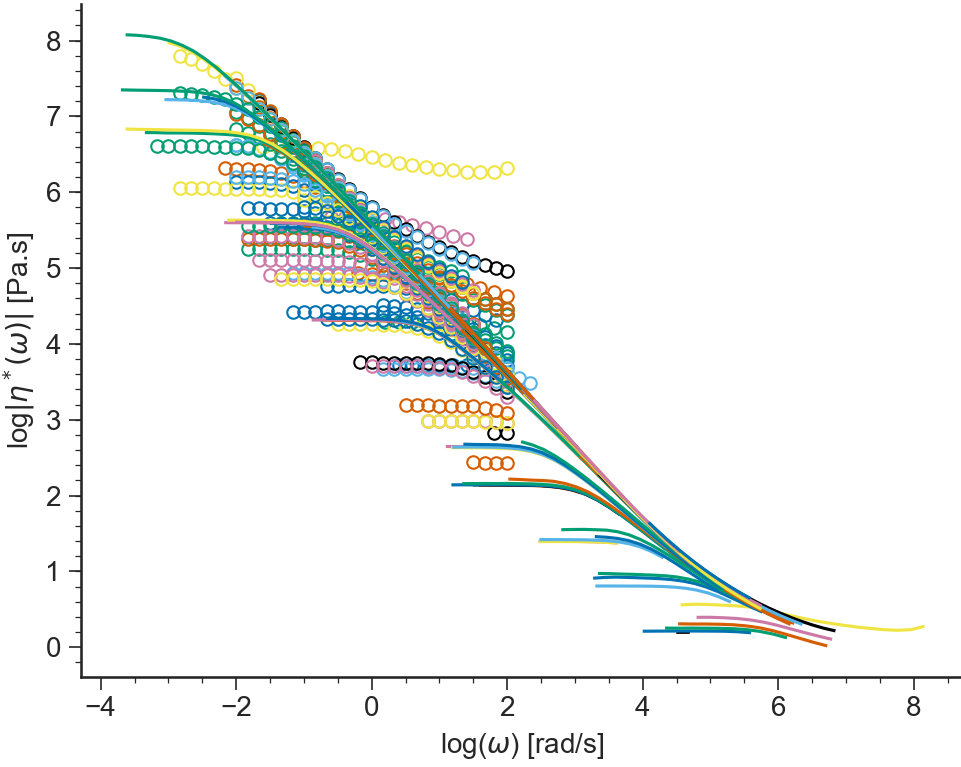### delta¶

BaseApplicationTTS.viewDelta()[source]

Loss or phase angle $$\delta(\omega)=\arctan(G''/G')\cdot 180/\pi$$ (in degrees, in logarithmic scale) vs $$\omega$$ (in logarithmic scale)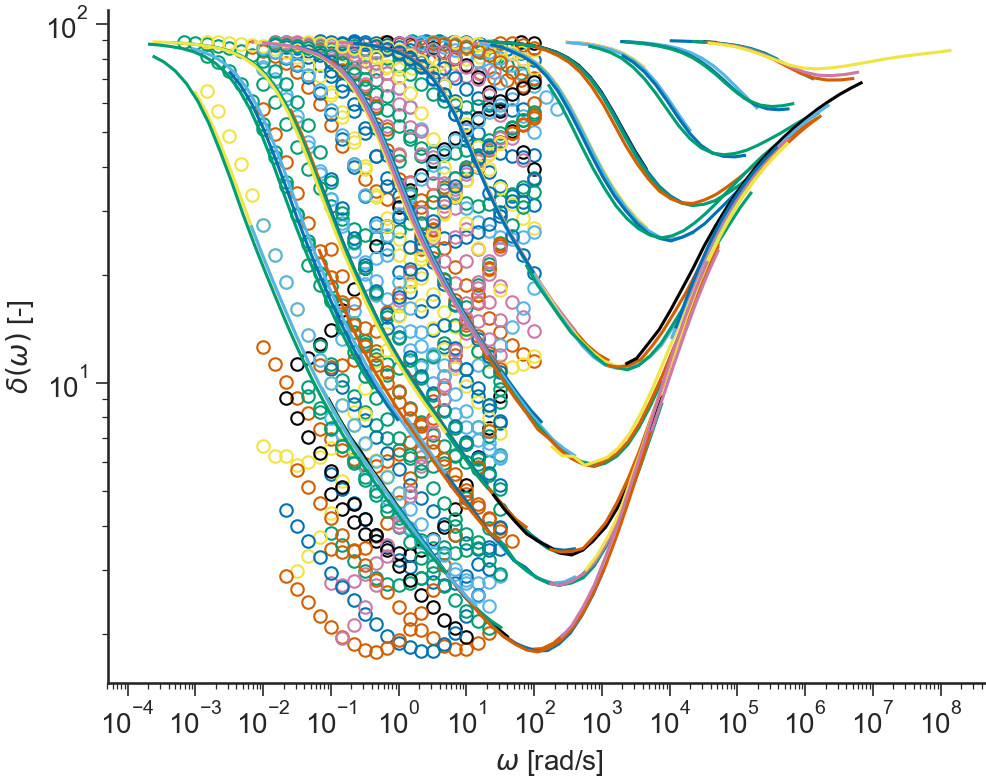### tan(delta)¶

BaseApplicationTTS.viewTanDelta()[source]

Tangent of the phase angle $$\tan(\delta(\omega))=G''/G'$$ (in logarithmic scale) vs $$\omega$$ (in logarithmic scale)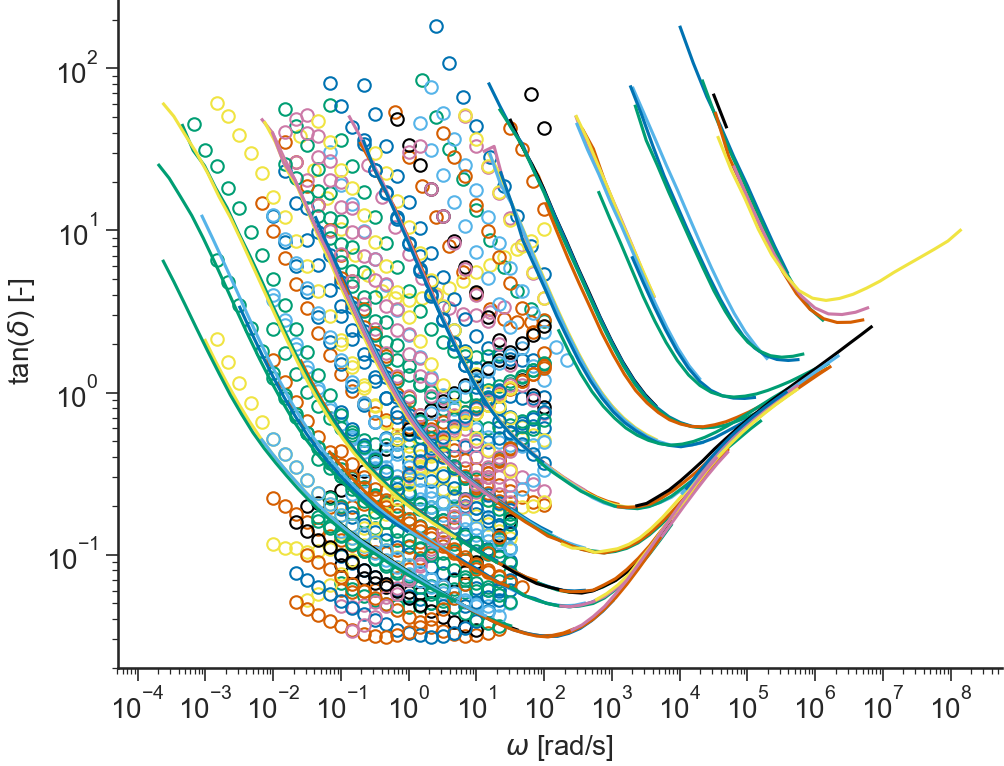### log(tan(delta))¶

BaseApplicationTTS.viewLogTanDelta()[source]

$$\log(\tan(\delta(\omega)))=\log(G''/G')$$ vs $$\log(\omega)$$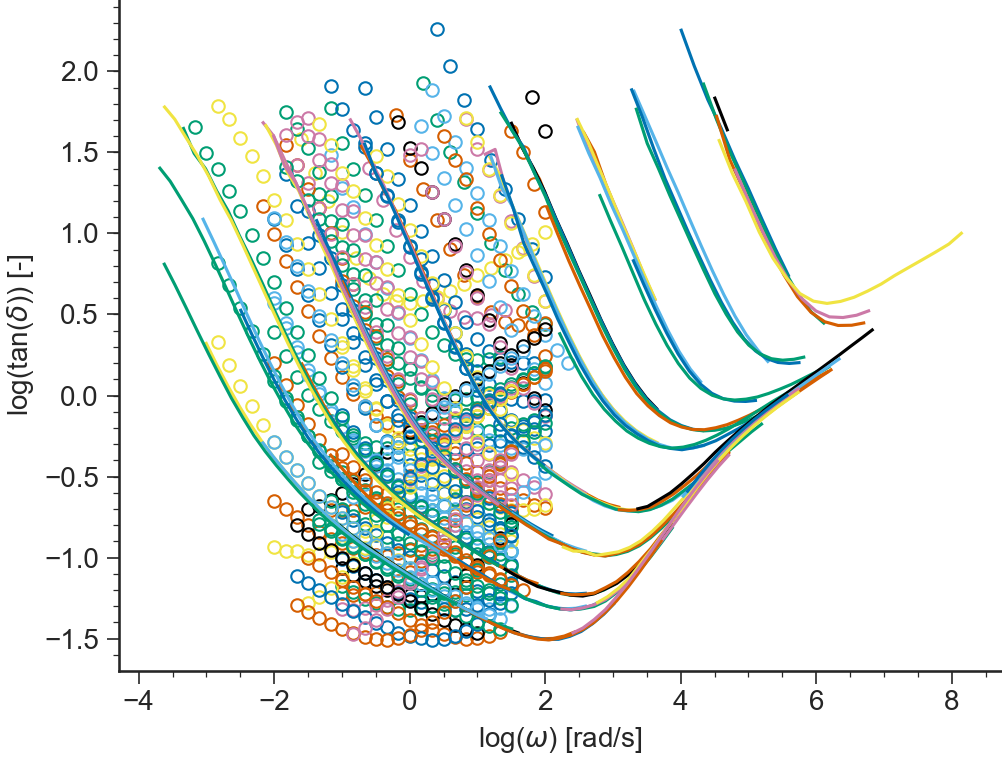### log(G*)¶

BaseApplicationTTS.viewLogGstar()[source]

Logarithm of the modulus of the complex viscosity $$|G^*(\omega)|=\sqrt{G'^2+G''^2}$$ vs $$\log(\omega)$$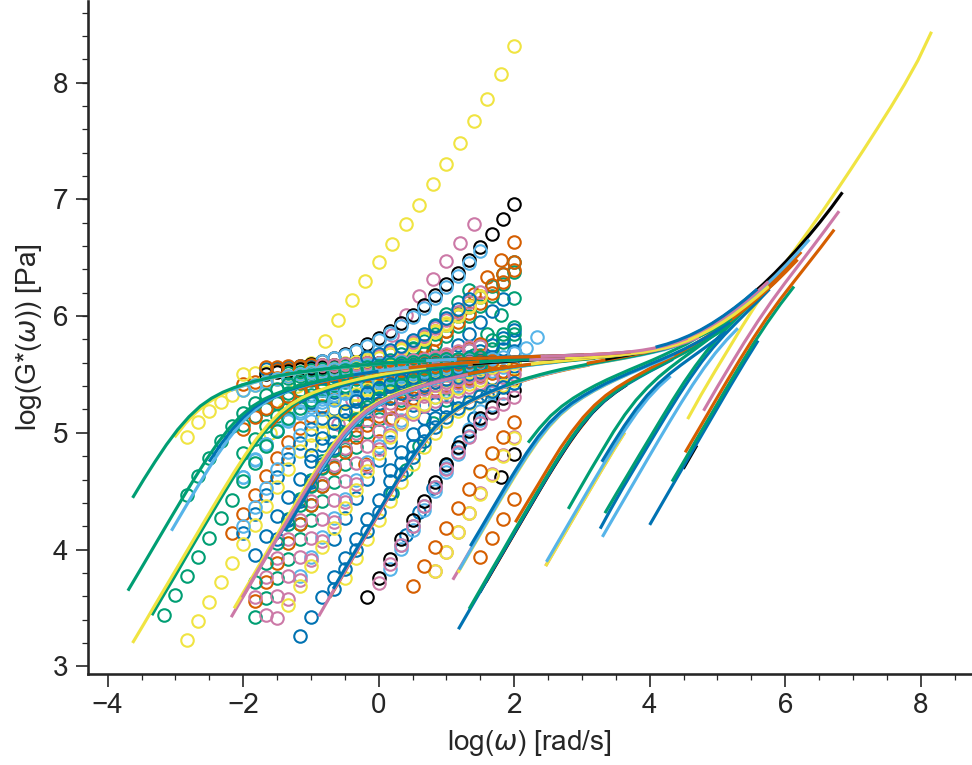### log(tan(delta),G*)¶

BaseApplicationTTS.viewLogtandeltaGstar()[source]

Logarithm of the tangent of the loss angle $$\tan(\delta(\omega))=G''/G'$$ vs logarithm of the modulus of the complex viscosity $$|G^*(\omega)|=\sqrt{G'^2+G''^2}$$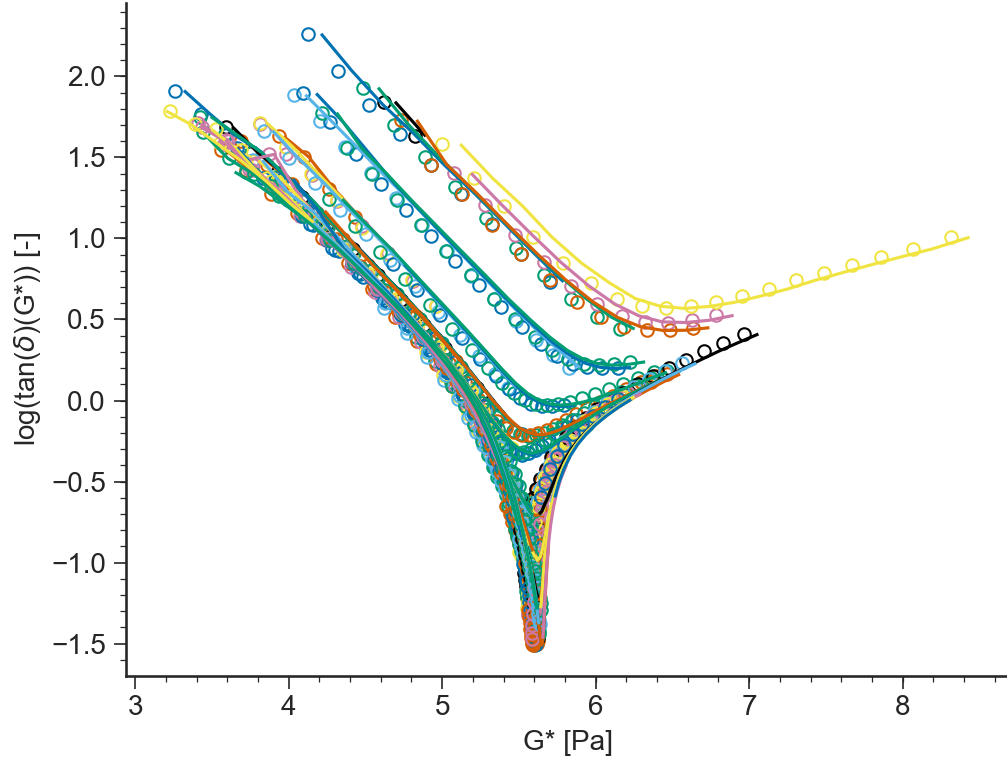### delta(G*)¶

BaseApplicationTTS.viewdeltatanGstar()[source]

Loss angle $$\delta(\omega)=\arctan(G''/G')$$ vs logarithm of the modulus of the complex viscosity $$|G^*(\omega)|=\sqrt{G'^2+G''^2}$$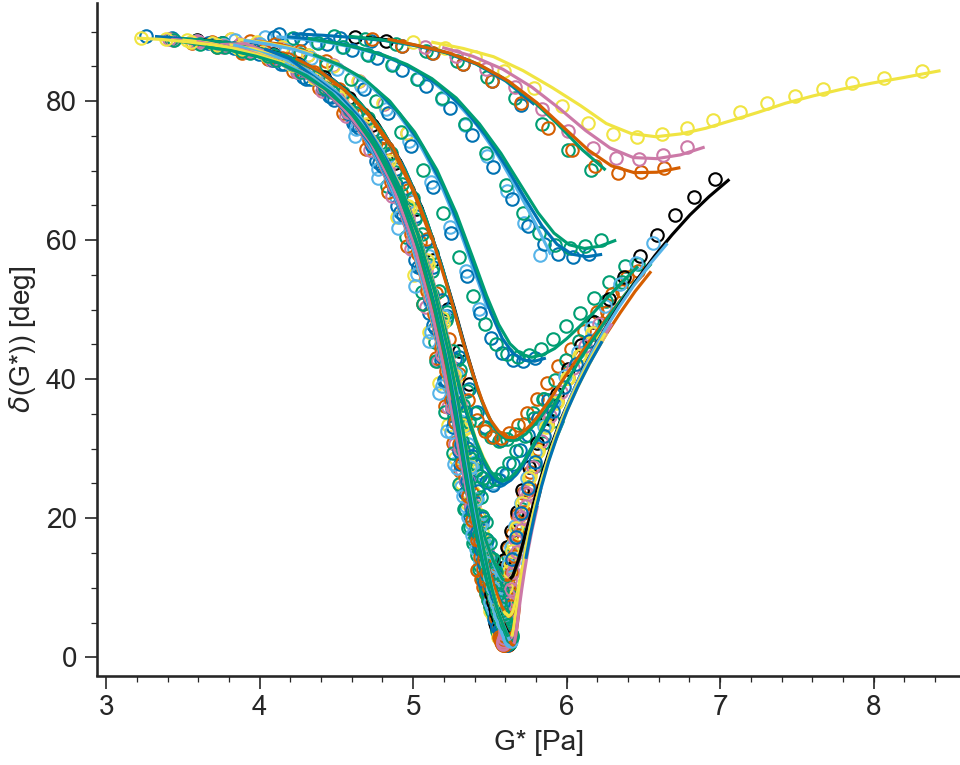### J’,J”(w)¶

BaseApplicationTTS.viewJ1J2()[source]

Storage compliance $$J'(\omega)=G'/(G'^2+G''^2)$$ and loss compliance $$J''(\omega)=G''/(G'^2+G''^2)$$ (in logarithmic scale) vs $$\omega$$ (in logarithmic scale)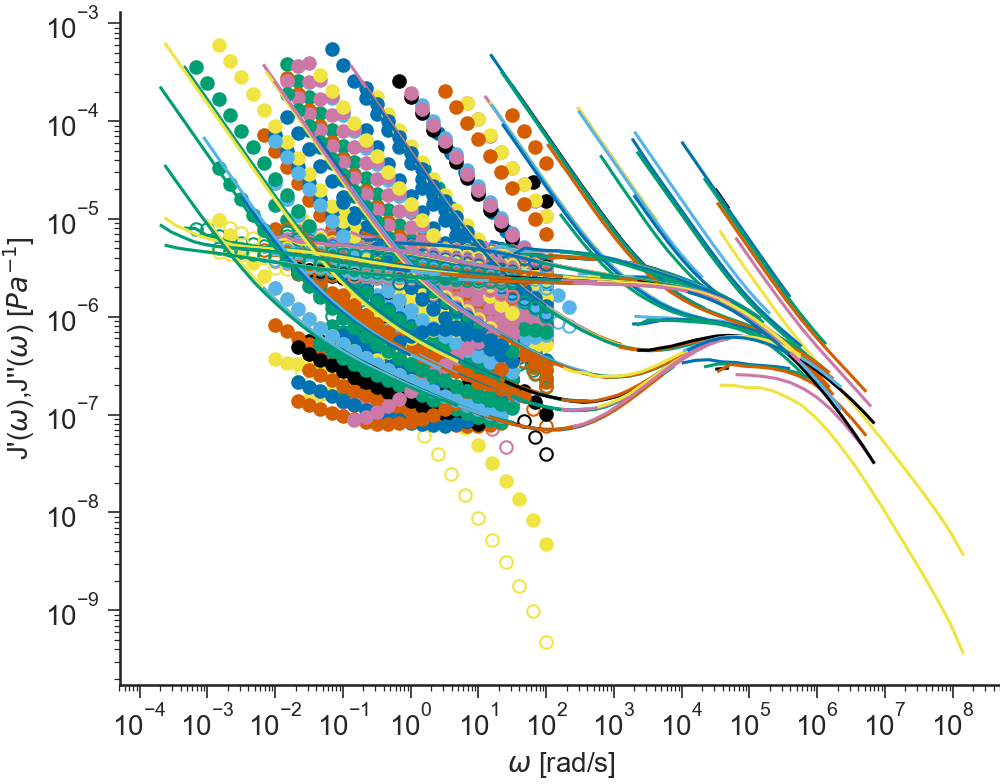### Cole-Cole¶

BaseApplicationTTS.viewColeCole()[source]

Cole-Cole plot: out of phase viscosity $$\eta''(\omega)=G'(\omega)/\omega$$ vs dynamic viscosity $$\eta'(\omega)=G''(\omega)/\omega$$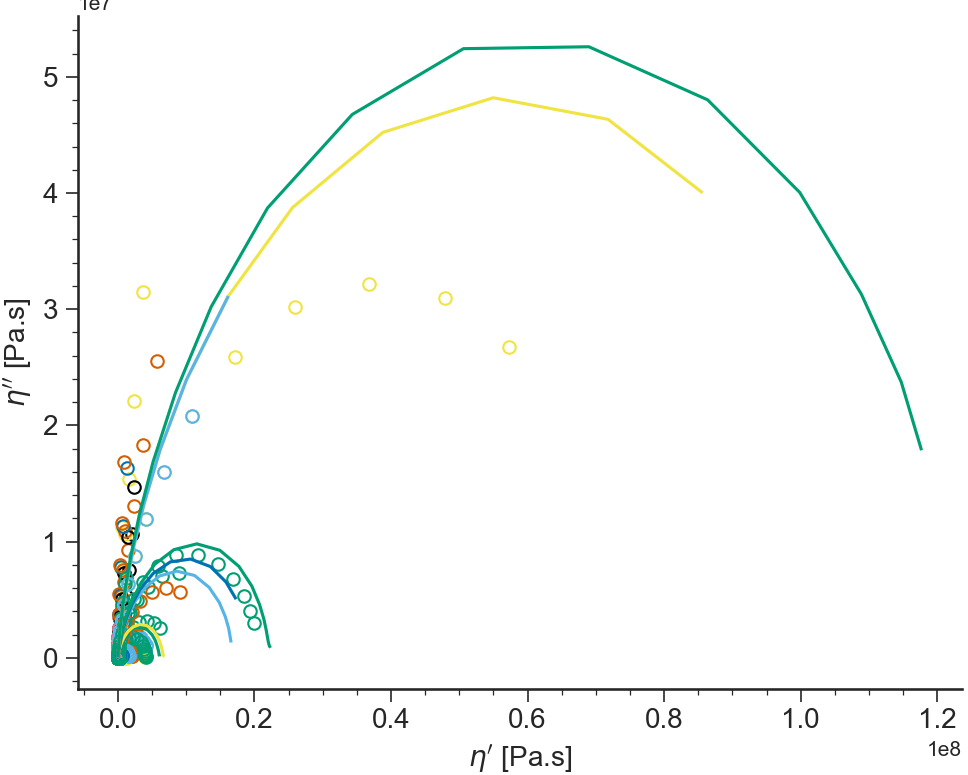### log(G’)¶

BaseApplicationTTS.viewLogG1()[source]

Logarithm of the storage modulus $$\log(G'(\omega))$$ vs $$\log(\omega)$$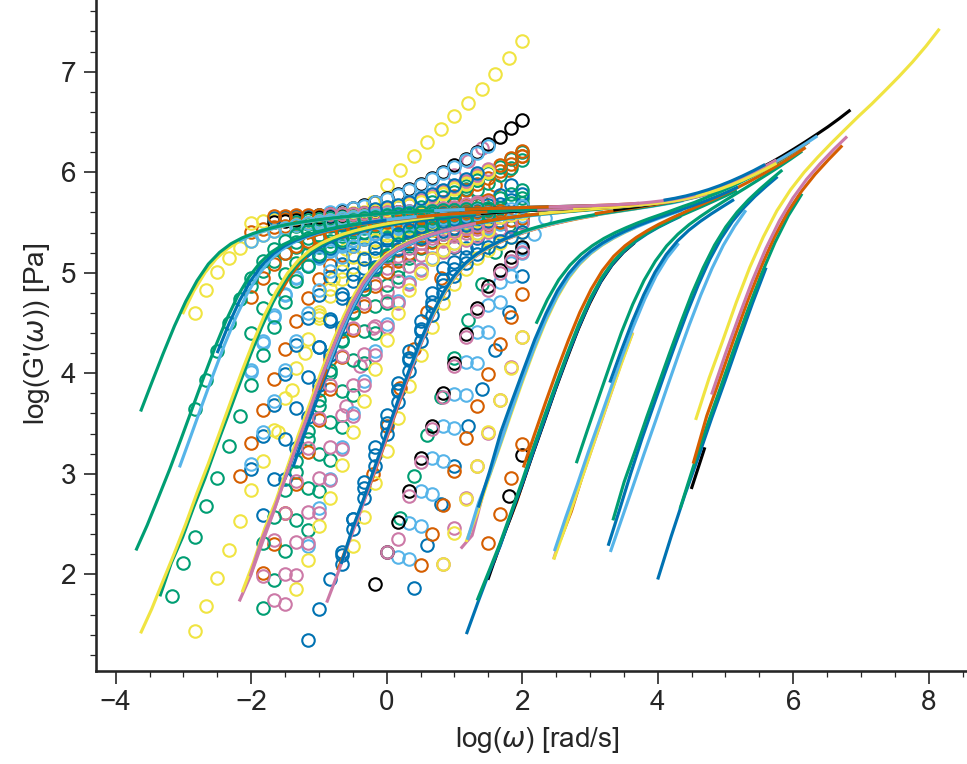### G’¶

BaseApplicationTTS.viewG1()[source]

Storage modulus $$G'(\omega)$$ (in logarithmic scale) vs $$\omega$$ (in logarithmic scale)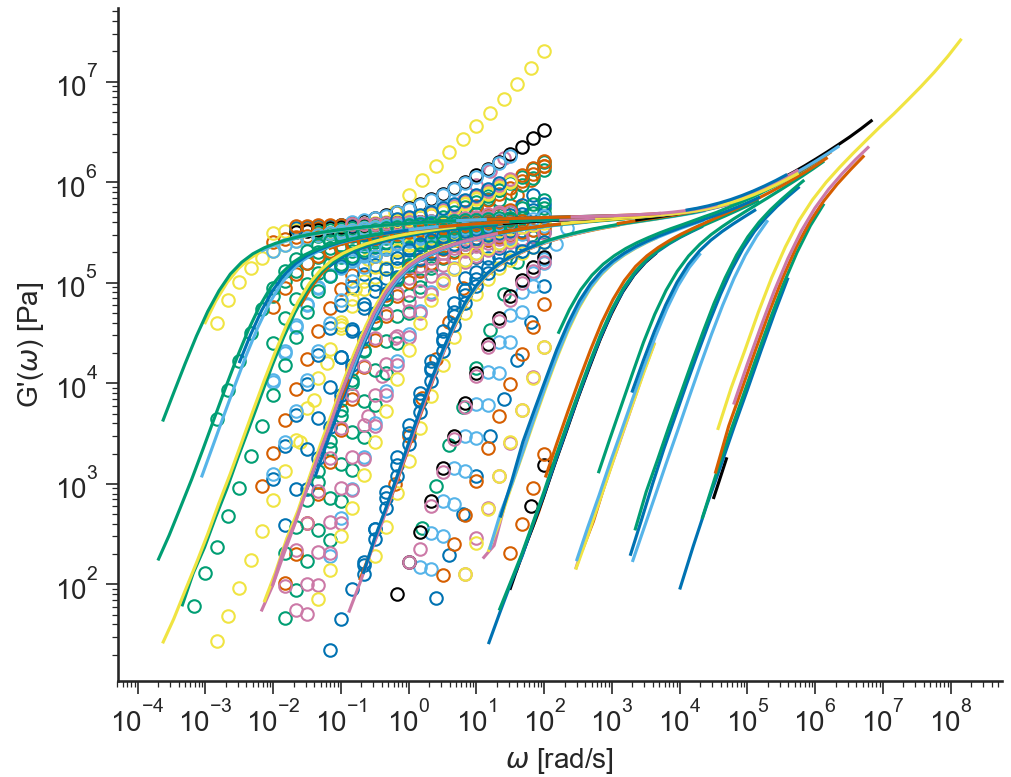### log(G”)¶

BaseApplicationTTS.viewLogG2()[source]

Logarithm of the loss modulus $$\log(G''(\omega))$$ vs $$\log(\omega)$$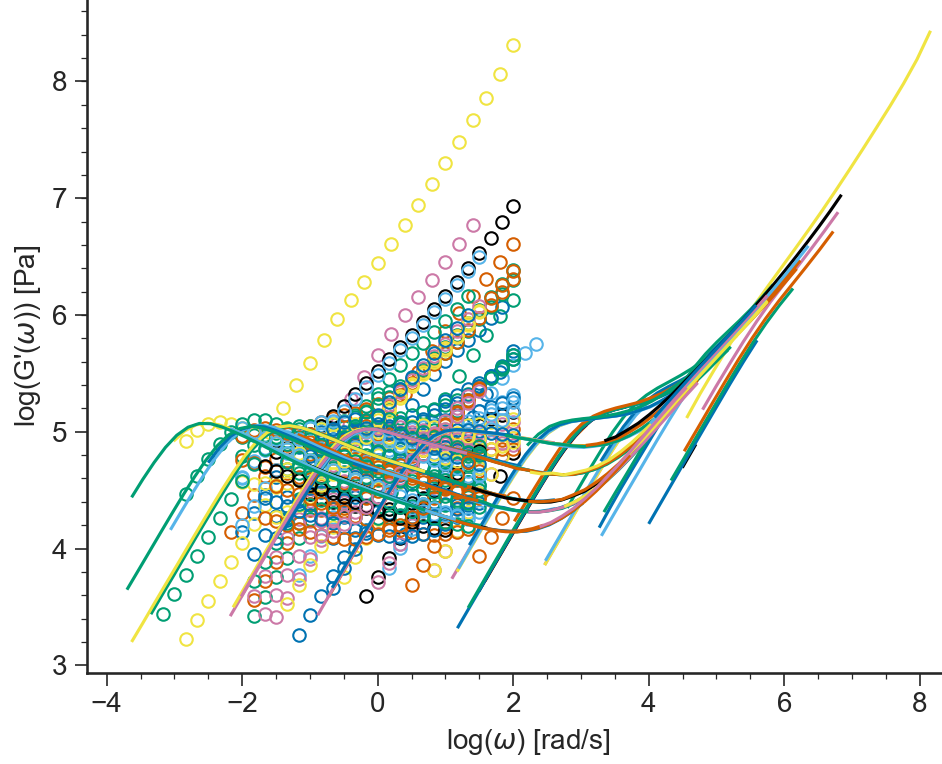### G”¶

BaseApplicationTTS.viewG2()[source]

Loss modulus $$G''(\omega)$$ (in logarithmic scale) vs $$\omega$$ (in logarithmic scale)### log(G’,G”(w),tan(delta))¶

BaseApplicationTTS.viewLogG1G2tandelta()[source]

Logarithm of the storage modulus $$\log(G'(\omega))$$, loss modulus $$\log(G''(\omega))$$ and tangent of the loss angle $$\log(\tan(\delta(\omega)))=\log(G''/G')$$ vs $$\log(\omega)$$